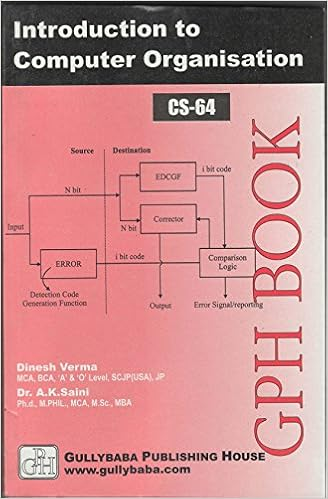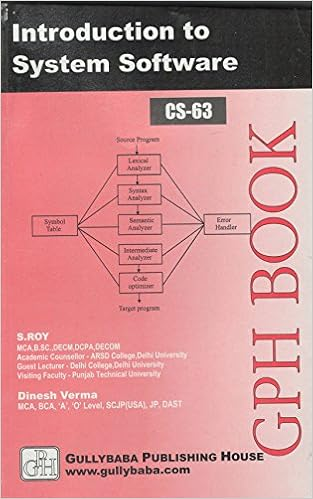IGNOU BCA MCA.comQuestion Papers of IGNOU MCA-NEW MCS013

 MCS013: Discrete MathematicsYear: 2005 TEE: December Time 2 Full Marks 50 Note: Question 1 is compulsory. Answer any three from the rest.Q.1(a): Wrtie the negation of the following statements: (i) For all x, x2 < x.(ii) There exists x such that x2 = 2. Q.1(b): Construct the cicuit that produces the follwoing output: x'/ (y / z')': Q.1(c): Let A = {1,2,3,4,5}. Construct a relation R from A to A such that R is reflexive and symmetric but not transitive': Q.1(d): Prove by induction that n3 - n is divisable by 3 for all positive integers.: home || Next >>